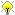[Documentation] [TitleIndex] [WordIndex]Please ask about problems and questions regarding this tutorial on answers.ros.org. Don't forget to include in your question the link to this page, the versions of your OS & ROS, and also add appropriate tags.

# Numerical Limits

Description: Fixing a few rough edges around c++ numeric limits.

Keywords: ecl numeric limits

Tutorial Level: INTERMEDIATE

This extends c++'s numeric_limits class for fundamental types, providing in addition, static constants that can be utilised as template arguments (as opposed to c macros or c++ functions in numeric_limits). For integral types, an example of the extras available:

```   1 ecl::uint16 bits = NumericLimits<int>::bits;
2 ecl::uint16 bytes = NumericLimits<int>::bytes;
3 int j = NumericLimits<int>::one;
4 int i = NumericLimits<int>::minimum;
5 int k = NumericLimits<int>::maximum;
```

An example of some extras for a float type:

```   1 ecl::uint16 bits = NumericLimits<float>::bits;
2 ecl::uint16 bytes = NumericLimits<float>::bytes;
3
4 // precision setting is a rough, but useful aid to bounding the accuracy for some math calculations
5 NumericLimits<float>::Precision p = NumericLimits<float>::dummy_precision();
6
7 // note, numeric_limits<float>::min() is the smallest
8 // positive value, this is the smallest negative value.
9 float minimum = NumericLimits<float>::minimum;
10 float maximum = NumericLimits<float>::maximum;
```

Note that it inherits the numeric_limits class, so all the functions therein can also be used.

2020-01-25 12:37